Fractions - math word problems - page 8

1. Balls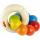The boys changed stamps, beads and balls. For 9 balls is 3 stamps, 2 balls is 44 stamps. How many beads is for 1 ball?
2. The balls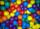You have 108 red and 180 green balls. You have to be grouped into the bags so that the ratio of red to green in each bag was the same. What smallest number of balls may be in one bag?
3. Grandmother's clocks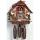Grandmother's clock is late every hour by half a minute. Grandmother it by set exactly at 8.00 am. How many hours will show after 24 hours.?
4. Decimal to fractionWrite decimal number 8.638333333 as a fraction A/B in the basic form. Given decimal has infinite repeating figures.
5. Stock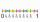Enterprise sold 7/12 of their products to foreign markets and 2/5 of the remainder sold at home. How many % of the products is still in stock?
6. Interest p.a.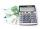Calculate the value of interest in period of 1 year from the deposit 62000 CZK at 8 percent interest rate, after deducting 15 percent tax.
7. Ratio of two unknown numbersTwo numbers are given. Their sum is 30. We calculate one-sixth of a larger number and add to both numbers. So we get new numbers whose ratio is 5:7. Which two numbers were given?
8. PipeSteel pipe has a length 2.5 meters. About how many decimetres is 1/3 less than 4/8 of this steel pipe?
9. Three men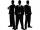Alex is half younger than Jan, which is one-third younger than George. The sum of their ages is 48. How are these three men old?
10. Concrete tank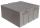How many m3 of concrete is in the tank shape of a cuboid with dimensions of 2000 cm and 5.6 meters and 70 dm, if the concrete takes up 3/7 of the tank?
11. Rope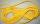Peter has a long rope 2 4/5 meter. Martin has a rope 20 cm longer. What distance ropes achieved if the boys link ropes?Mom bake cookies. Rolo took 2/9 of all cookies, Michal 3/9. How many cookies ate Rolo if Michal had 9.
13. Cake fractions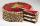Thomas ate 1/3 of cake, Bohouš of the rest of the cake ate 2/5. What fraction of cake left over for others?
14. Boots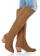Master shoemaker has three apprentices. First do one pair of boots for two days, second for 1 day, third on 1.5 day. If they worked together, for how long it would take made a couple of boots?
15. Minutes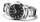Write as fraction in basic form which part of the week is 980 minutes.
16. President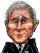The country has 5.11 million citizens which 2.12 million are voters. Turnout was 34.4%. President R. F. was elected from 20 candidates for president and won with 38% of votes. Calculate what percentage of the country's citizens voted R.F. as president o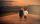Peter and Franta threw to the basket. Each had 20 attempts. Peter scored thirteen and twelve Franta. Give them success in percentage.
18. Playing Cards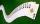Kara has 2 times more cards than Dana, Dana has 4× less than Mary. Together they have 728 cards. How many cards has each of them?
19. Master and apprentice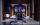Master painted the roof in 3 hours and apprentice for 4 hours. How many of roof they painted in hour and how many in three quarters of an hour?
20. The rod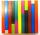The rod is painted in four colors. 55% of the bar is painted in blue, green 0.2 of rod, 1/8 is brown and the remaining 45 cm of white. How long is rod?

Do you have an interesting mathematical word problem that you can't solve it? Enter it, and we can try to solve it.

To this e-mail address, we will reply solution; solved examples are also published here. Please enter e-mail correctly and check whether you don't have a full mailbox.

Need help calculate sum, simplify or multiply fractions? Try our fraction calculator.